# Force And Law’s of Motion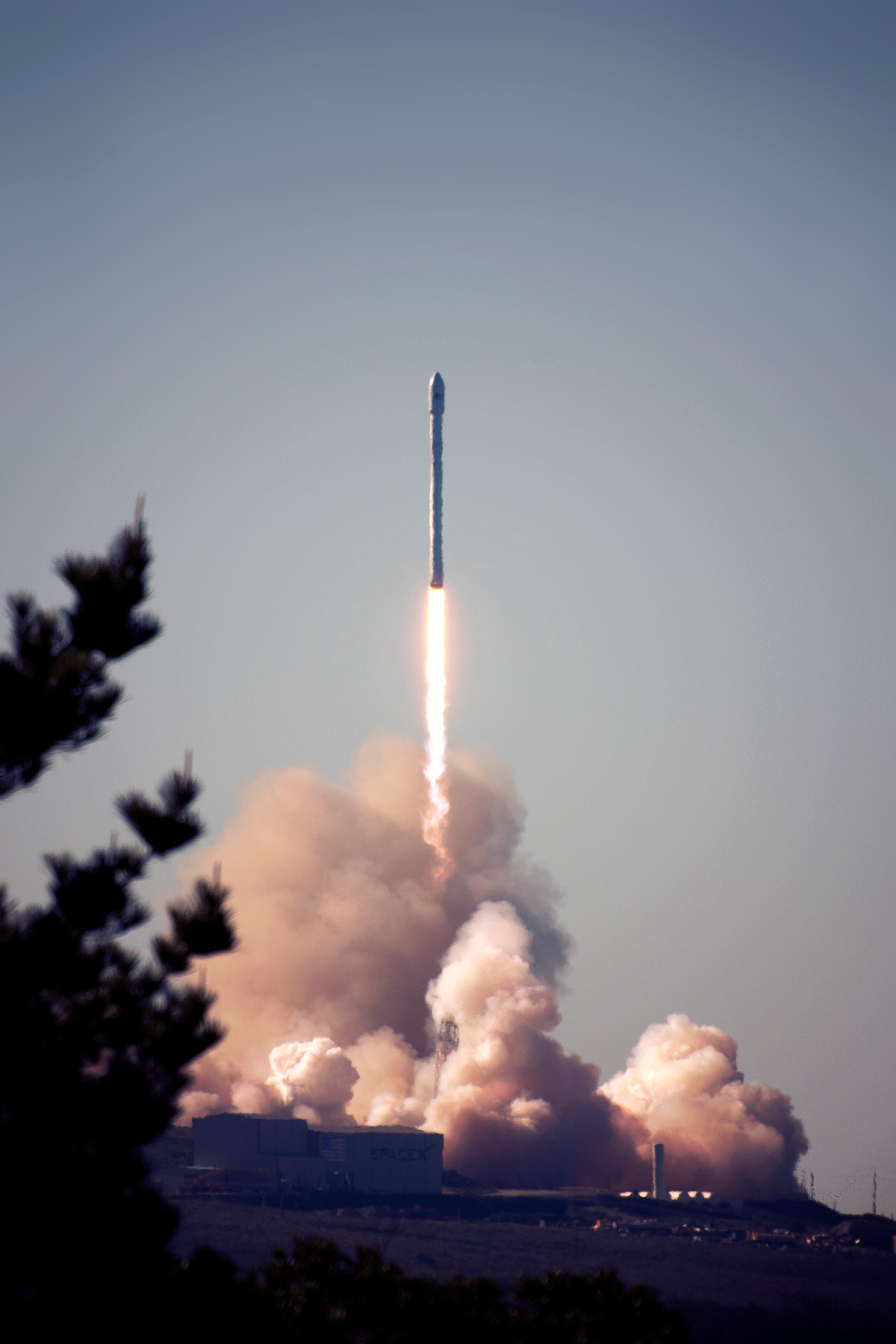Photo by Tim Mossholder on Unsplash

When we want to open the door and close the door, pick up any objects in his hand, pull the branch to move it from its position, push the basket on the wall side. In all situations, we either pull or push the body to move it from its earlier position. These are some examples, but in our everyday life, all objects move by this pull and push functions. . Our everyday life, all objects move by this pull and push functions, So we can say this all objects move by the apply of Force on it. Force is used in our everyday actions like . We know, to move any object we used force on it. So, Force has some characteristics that move any stationary objects. Let’s discuss these effects of force.

Effects Of Force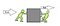Take from Byju’s

All force be not always equal. Some force (that act on a body) very greater ( ) that moves the body. But, Some time force is not so greater and , So this force does not move the body. We see some time force small or sometimes large and sometimes it moves the body, but sometimes not. Let’s discuss, Why this happened.

## Balanced Forces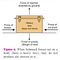From Lakhmir sign

In this picture, Four different types of forces applied to the body. These forces are the (that oppose the force of our push), the and. These forces are balanced force because the force of friction balances the force of our push and force exerted by the ground balance the force of gravity. So, all force has canceled and has been . Due to this reason, the box cannot move from its position ( ).

## Unbalanced Forces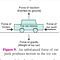From Lakhmir sign

In this picture, again four different types of forces applied to the car. Again, Force exerted by the earth balances the force of gravity (). But that time, the force of our push greater than the force of friction (). So, Force of friction cannot balance the force of our push. Now all the forces acting on the car are not Zero (). Due to this reason, the car may move in the direction of greater force(), and the direction of our push in the forward direction. So, the car move in the forward direction.

Conclusion of the above discussion, Due to unbalanced force all the bodies move in our surrounding if no unbalanced force exist than movement is not possible.

# Newton’s Law Of Motion

In the seventeenth century a “natural philosopher” name Sir Isacc Newton. He was given three laws of motion. He has described these laws in the “Principia Mathematica” a book written by Newton own. Newton did not give these laws directly, first, he studies the law of Galileo Galilee.

Galileo’s Law Of Motion

Galileo’s law also called . Newtons’ 1st law and Galileo’s law some similar. But why Newtons’ 1st law so famous and Galileo’s law not. Let’s studies about Newton, 1st law. And try to find the answer to it.

## Newton’s First Law Of Motion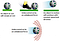credit #MyRank

Newton said first, a body that stands in the rest state will always stand in the rest position. Unless an unbalanced external for applied on them. When an unbalanced external for act on them then the leave his rest state and move in the direction of external force.

Second, a body that travels with uniform motion always travels in the straight-line direction with the same uniform motion (constant motion). Unless an unbalanced external for applied on them. When an external force act on them .

Galileo said the only “the second statement of Newton’s 1st law.” But newton also said this, a body stand-in rest remain in the rest unless an unbalanced external for applied on them.

We study the present in which position will continue in that always when no unbalanced external force act on them. That means something is present that made the body continue in there last position. What is this? This is called .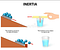From Quora#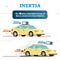# Pinterest

Inertia is proportional to the mass of the body. So, If the mass of the body higher (leather ball) then inertia is large, and if the mass is low (plastic ball) then inertia is small.

## Newton’s Second Law Of Motion

Now one question is arises from the above statement. And this is a very important term to understand this law and force.
What is Momentum? Momentum is a term which has given by .

The symbol of momentum is . The SI ( standard identity) unit of momentum is Momentum is a

Force is proportional to the rate of change of momentum F∝(mv-mu)/t. And force is the product of two terms Mass and Acceleration.
If force will increase than the acceleration of the body will also increase, and if force decrease than the acceleration of the body will decrease. And if the mass of an object is higher then we need to apply more force on them for made them move, and if the mass is low then we need to apply low force to move them. So,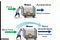credit #brainly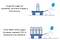# Tes tech

Suppose an object of mass () is moving along a straight line with an initial velocity (). It is uniformly accelerated to velocity () in time () by the application of a constant force () throughout the time(). The initial and final momentum of the object will be p1 = and p2 = respectively.

The change in momentum ∝ p2 — p1
The change in momentum ∝ mv — mu
The change in momentum ∝ m × (v — u).

And the rate of change of momentum ∝ m × (v-u)/t

Force is proportional to the rate of change of momentum. So

Force ∝ the rate of change of momentum
Force ∝ m × (v-u)/t
Force = m × (v-u)/t

Acceleration is equal to (initial velocity — final velocity)/time taken so

F = m × a
F = m a

The symbol of the Force is F. And the SI unit of Force is Newton. Force is the.

One other term’s name comes from the rate of change of momentum. By it, we also find the change in the momentum in the given interval of time.

F = m a

Replace the to the full expression of acceleration:

F = m ×(v-u)/t
F = (mv-mu)/t
Ft= mv-mu

J (Imp) = Ft

The symbol of impulse isThe SI unit of it is .

When we are not applying any external force on the body (F= 0 N) for any interval of time (t).
Then the change in momentum = 0 N × t = 0. So, the change in momentum is 0 means initial momentum (mu) = final momentum (mv). So, if the body travel in uniform motion will continue in the uniform motion and if the body in rest state will remain in the rest position.

## Newton’s Third Law Of Motion

The action and reaction are the same in the magnitude. But mostly, they not produced an equal magnitude of acceleration in both bodies, Because mostly condition the mass of the two bodies is different from each other. But if the mass of both has the same then the magnitude of the acceleration is the same.

When one body exerts some force on the other body like when we walk on the road then we exert force on the road in the backward direction (), then the road exerts an equal and opposite force (in the forward direction) on us (), that means the second body immediately exert an equal and opposite on the first one. By this process, we walk in the forward direction.
The swimmer pushes the water in backward (), the water pushes the swimmer in the forward () and pushes her forward.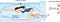From # Lumen Learning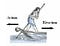Credit # brainly.in

See all the above example images and try to understand the Action and Reaction force of how to work.

## Conservation Of Momentum

Suppose two ball and of masses and are traveling in the same direction along a straight line at different initial velocities and , respectively. And no other external unbalanced forces are acting on them. Let > and the two balls collide with each other. During collision which lasts for a time t, the ball exerts a force on ball and ball exerts a force F_ba on ball . Suppose and are the final velocities of the two balls A and B after the collision, respectively.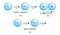# NCERT

Similarly,

According to the Newton Third Law Of Motion, So,

F_ab =-( F_ba)
mA (vA- uA)/t = -( mB ( vB — uB)/t )

Multiply to both side:

mA (vA- uA) = — mB ( vB — uB)
mA×vA-mA×uA =-mB×vB + mB×uB
-mA×uA -mB×uB = -mA×vA -mB×vB
-(mA×uA + mB×uB) = -(mA×vA + mB×vB)

Canceling (-) to both side:

mA×uA + mB×uB = mA×vA + mB×vB

As a result of this ideal collision experiment, we say that provided there is no external unbalanced force acting on them. This is known as the .